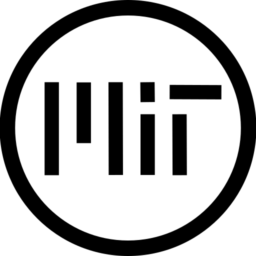# UCBC’s Stylized Light with Light Masking

A simple Light shader that uses an RGB ramp to interpolate the light values and use those interpolated values to Step the light and Mask the Shadows, Lights and Highlights on the model, this masked values can be used with a light texture for said Shadows, Light and Highlights to add extra detail if needed.

It also has a built in Outline however this can be disabled easily by commenting the USE_OUTLINE definition.

`IMPORTANT`

In order for the shader to work properly light_ramp needs a texture.
basically, RED is the shadow area, Green is regular light and Blue are for the highlights. the default Grayscaled GradientRamp1D will make the shader look off.

`HOW TO GET RESULTS LIKE THE SCREENSHOTS`

2. change the GradientRamp1D color space from sRGB to LinearRGB [ optional ]
3. change the first color to pure RED, and change the last color to pure BLUE
4. add a color in the middle and make that color pure GREEN
5. change interpolation mode from Linear to Cubic.
6. set the width to a low value, the lower the value the less transitions resulting in a step effect.
``````shader_type spatial;
render_mode vertex_lighting;

//Uncomment this to use the OUTLINE

#define USE_OUTLINE;

group_uniforms LIGHT;
uniform sampler2D light_ramp : hint_default_white,filter_nearest,repeat_disable;
uniform float normal_smoothness : hint_range(0.0, 1.0) = 0.1;

#if defined(USE_OUTLINE)
group_uniforms LIGHT.OUTLINE;
uniform vec3 outline_position = vec3(0.0,-0.25,-1.0);
uniform float normal_smoothness_outline : hint_range(0.0, 1.0) = 0.1;

//outline_edge_a and b are used to make the outline, thicker, thinner, smoother or harsher.
uniform float outline_edge_a = 0.5;
uniform float outline_edge_b = 0.5;
uniform vec4 outline_tone : source_color = vec4(0.0,0.0,0.0,1.0);
#endif

group_uniforms LIGHT.TEXTURES;
uniform vec2 light_uv_scale = vec2(1.0);

uniform sampler2D specular_texture : source_color, repeat_enable;
uniform sampler2D light_texture : source_color, repeat_enable;
uniform sampler2D shadow_texture : source_color ,repeat_enable;

group_uniforms LIGHT.TONES;
uniform vec3 highlight_tone : source_color = vec3(1.0);
uniform vec3 light_tone : source_color = vec3(0.75);
uniform vec3 shadow_tone : source_color = vec3(0.25);

float linearizeDepth(float _depth, float _near, float _far) {
return (2.0 * _near * _far) / (_far + _near - (_depth * 2.0 - 1.0) * (_far - _near));
}

void light(){
float depth = (linearizeDepth(FRAGCOORD.z,0.005,0.1) / 0.1);

//So, normal smoothness is an interpolation from the current NORMAL position to the LIGHT position
//so the light will be 100% visible no matter what.
vec3 lightNormal = NORMAL;
lightNormal.rgb = mix(lightNormal.rgb,LIGHT,normal_smoothness);

//LNDotL stands for LightNormal Dot Light, is just so we know how much light that part is facing.
float LNDotL = clamp(dot(lightNormal,LIGHT),0.0,1.0) * ATTENUATION;

vec3 light_map = texture(light_ramp,vec2(LNDotL,1.0)).rgb;
vec3 light = light_tone * textureLod(light_texture,UV * light_uv_scale,0.0).rgb;

#if defined(USE_OUTLINE)
//WE store the Raw Normal, we unpack it, and we smooth it out based on a our factor
vec3 outlineNormal = NORMAL;
outlineNormal = outlineNormal * 2.0 - 1.0;
outlineNormal.rgb = mix(outlineNormal.rgb,LIGHT,normal_smoothness_outline);
//We make the same formula for the light intentsity, but using a custom position for a FakeLight to create a mask
float outline_map = clamp(dot(outlineNormal,outline_position),0.0,1.0);
outline_map = smoothstep(outline_edge_a,outline_edge_b,outline_map) * depth; //We use smoothstep so we can have thicker outlines, softer or harsher ones.
vec3 outline = outline_tone.rgb;
DIFFUSE_LIGHT += mix(light* LIGHT_COLOR,outline * LIGHT_COLOR,(outline_map * outline_tone.a) - light_map.b);
#else
DIFFUSE_LIGHT += light* LIGHT_COLOR;
#endif
SPECULAR_LIGHT += light_map.b * (textureLod(specular_texture,UV * light_uv_scale,0.0).rgb * highlight_tone);
}``````
###### Tags
3d, light, stylized, toonThe shader code and all code snippets in this post are under MIT license and can be used freely. Images and videos, and assets depicted in those, do not fall under this license. For more info, see our License terms.

### Stylized shadows, not a post processing

Subscribe
Notify of1 Comment
Inline Feedbacks